# Creative Coding — Generate a geometric figure with motion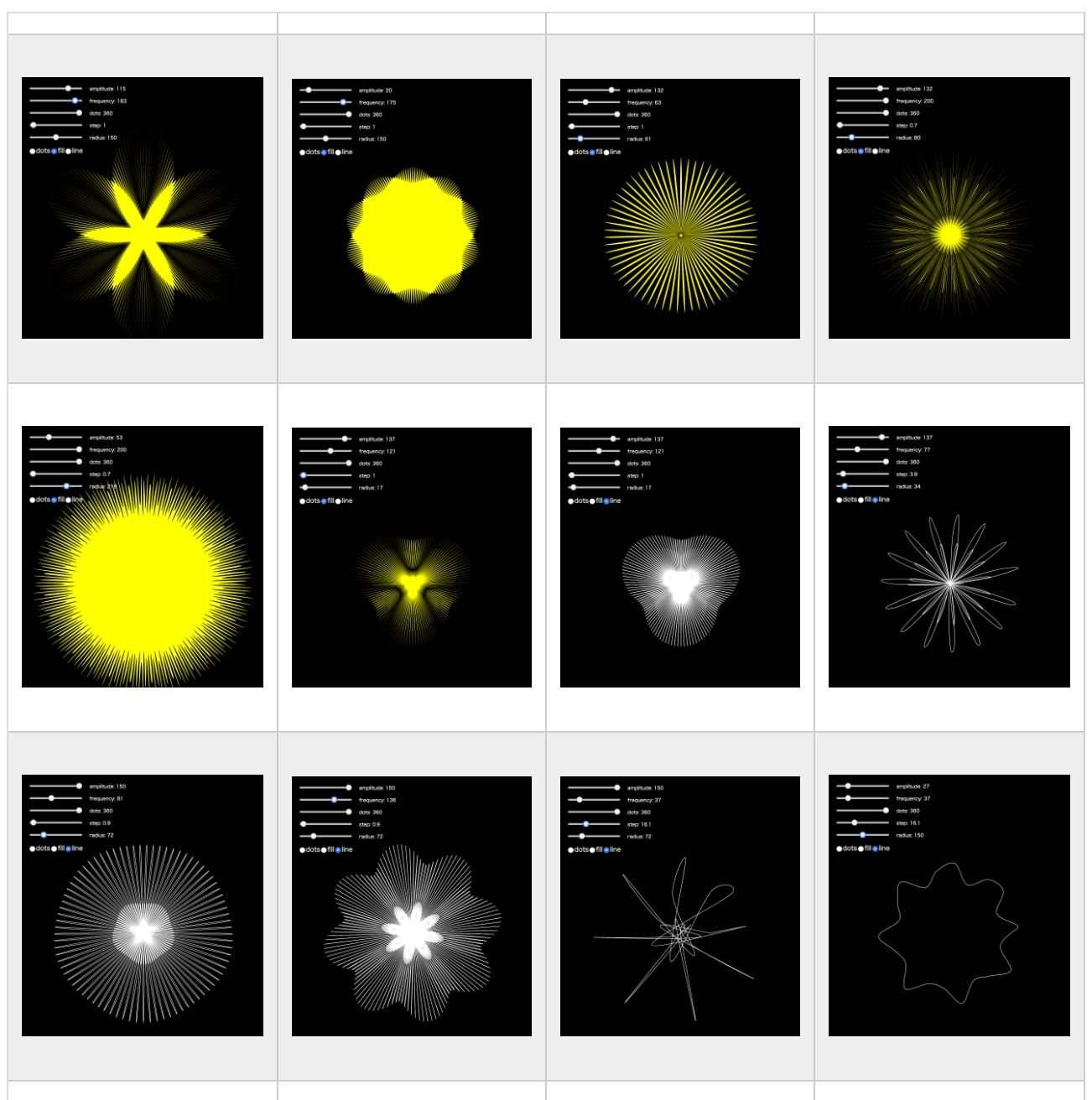Have you ever heard Wolfram Alpha, a search engine dedicated to computer science?I knew this WolframAlpha at a session of SXSW in 2017. I couldn’t, however, imagine indeed what the search engine is in that time. I forgot it since then, but recently I encountered the information again.

## What is Wolfram Alpha?

WolframAlpha seems to be a search engine that displays the results of mathematical queries. It is possible to search for natural language in the same way as Google search, but it does not mean to return documents or pages stuck in search. It is a mechanism to interpret the search contents mathematically and return the result with graphical image. Surprisingly Japanese is also available to input. For example, if “eight coin tosses” is input in the search box, the sentence is interpreted and the result is output as below.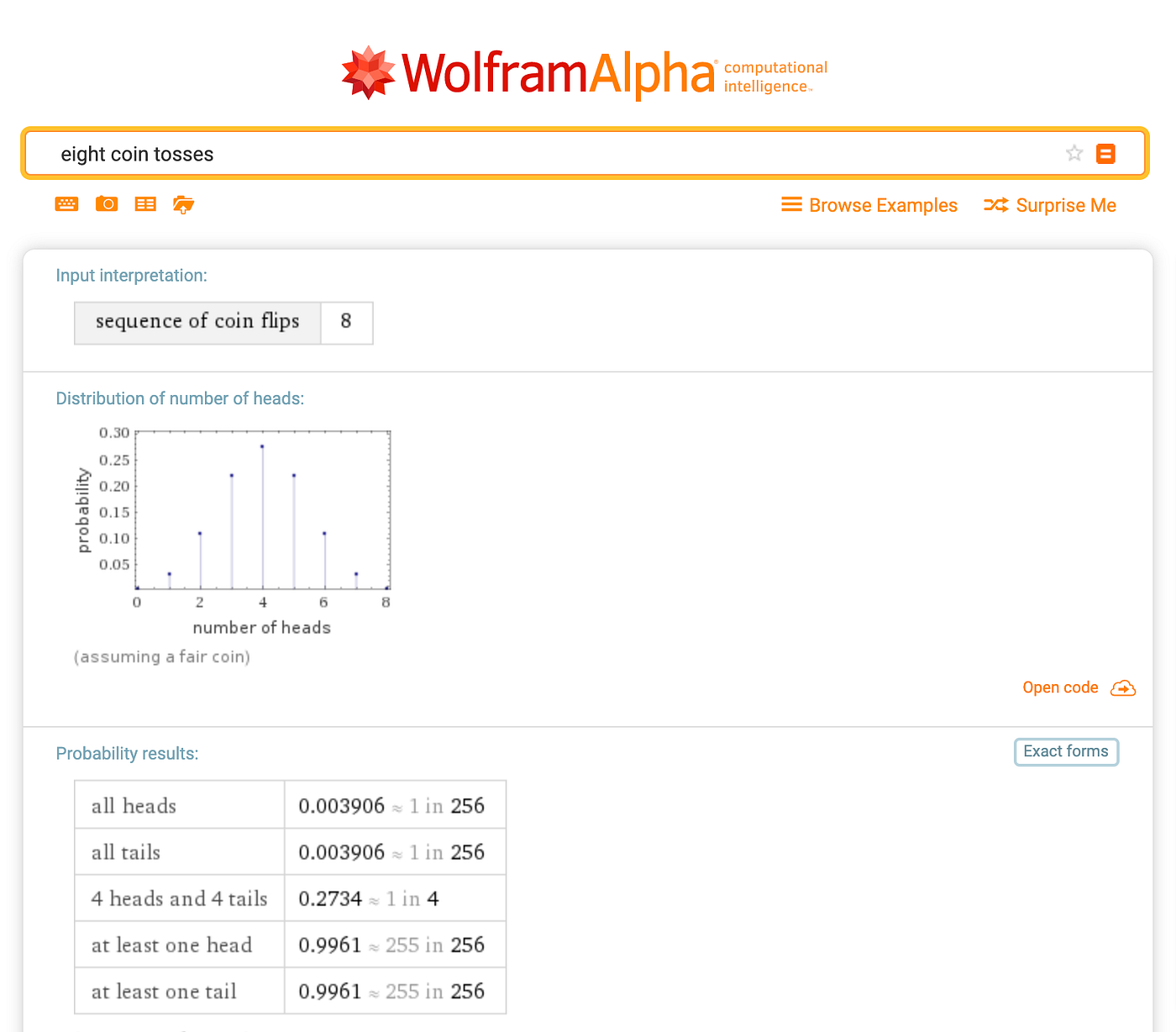This search engine allows you to search not only mathematic but also other topics as well as movies, arts, media, entertainment and so on. I tried to input “Star Wars franchise writer, director” and result is below.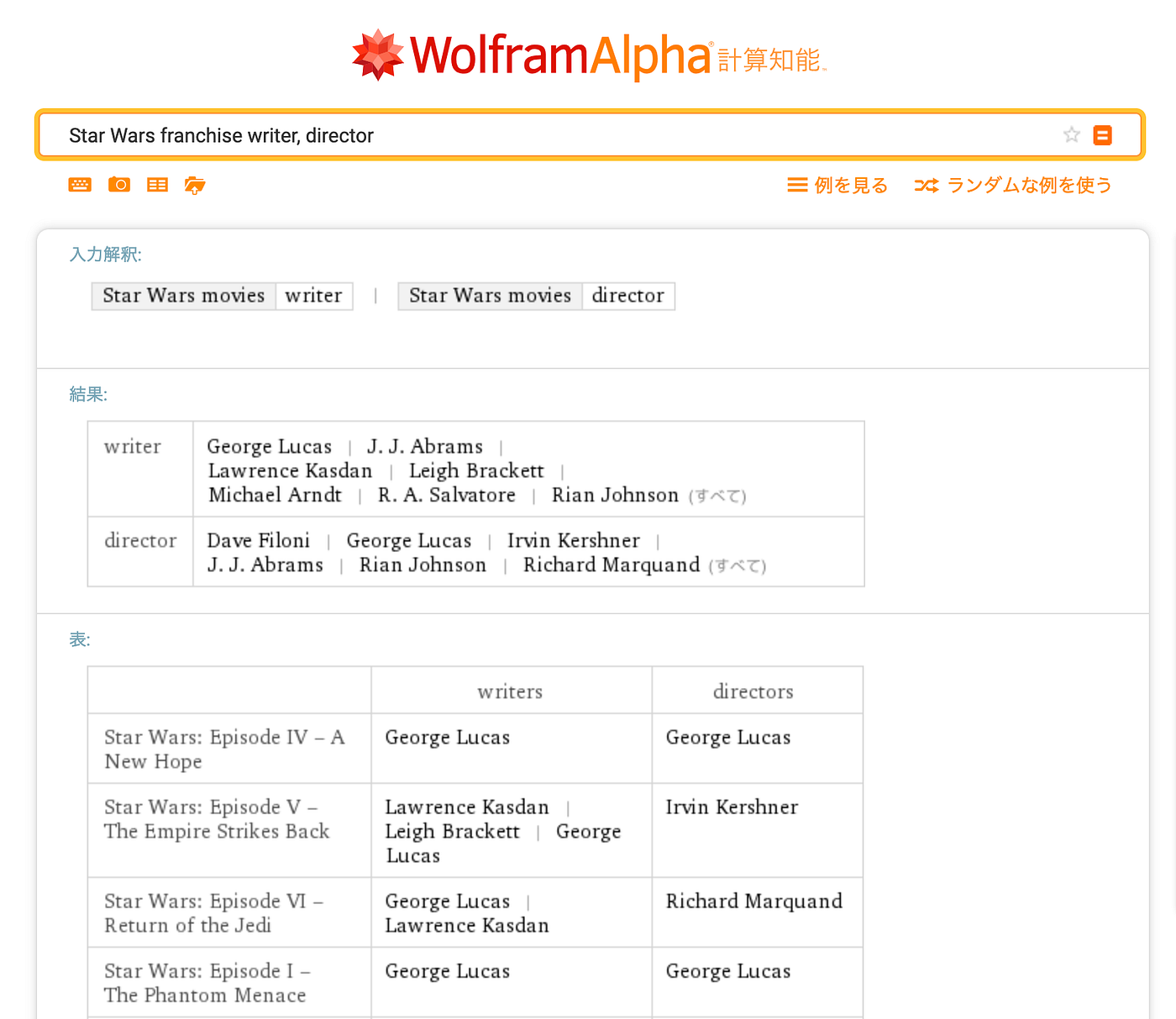## Interesting Figure

Using this search engine, I found strange but curious figure with custom sin formula. The following is the image. I tried to manage to draw the image by JavaScript.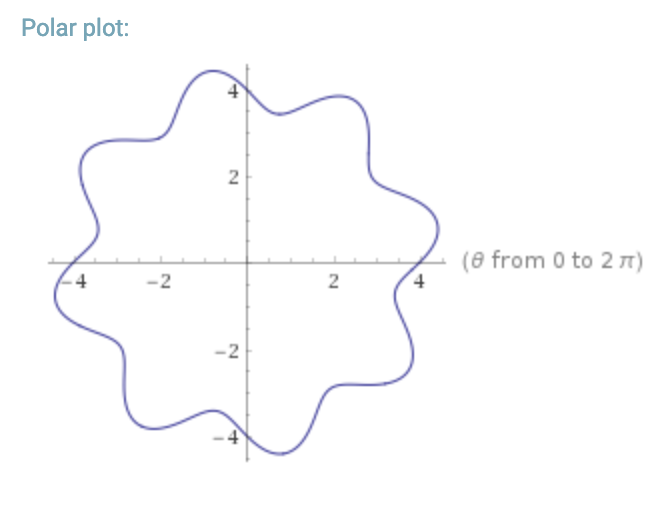# Fomula
r = 4 + 0.5 * sin( 8 theta)

It is an equation to calculate the radius R, but the circle is distorted according to the angle of the theta. I think the structure of this formula is as follows.

Radius = Size of Radius + Amplitude * sin( Frequency * theta)

Jot down above formula by JavaScript with p5.js.

// p5.js
const amplitude = 12;
const frequency = 12;
translate(width / 2, height / 2)
for (let i = 0; i < 360; i++){
const r = radius + amplitude * sin( radians(frequency * i ))
}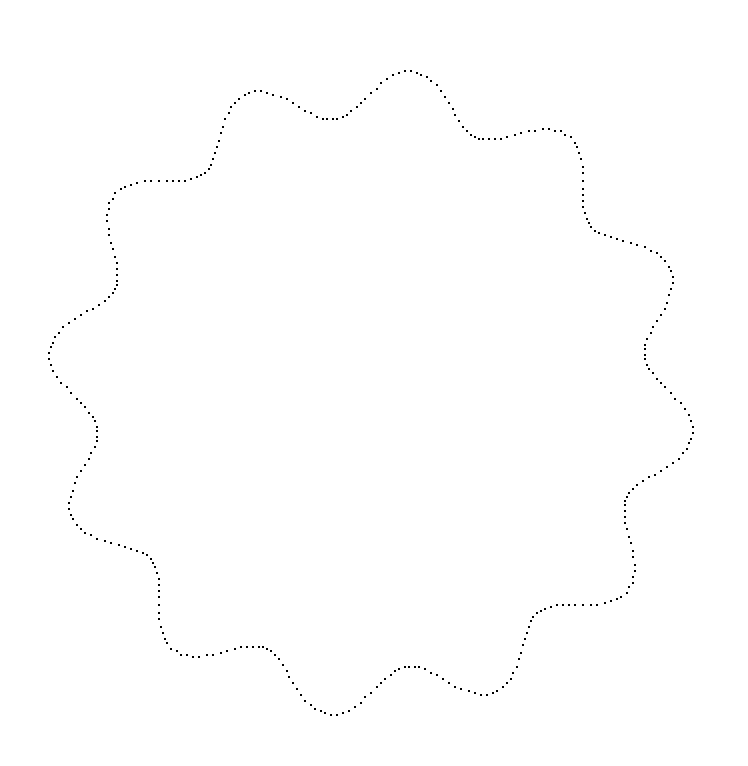Based on this basic principle, I made a generator to create a creature called Ameba. It may not be visible to Ameba. . . I leave it on GitHub. You can also try it on OpenProcessing.